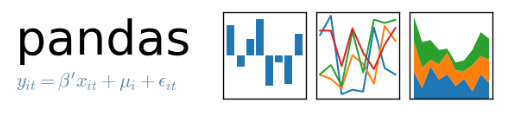[技术干货] 【Python算法】分类与预测——K近邻分类算法

# 【Python算法】分类与预测——K近邻分类算法

1.K-近邻算法（KNN）概述
最简单最初级的分类器是将全部的训练数据所对应的类别都记录下来，当测试对象的属性和某个训练对象的属性完全匹配时，便可以对其进行分类。但是不可能所有测试对象都会找到一摸一样的训练对象。也出现过将某一个训练对象分类为很多类别的情况。正是因为这些问题的产生，KNN诞生了。
KNN通过测量不同特征值之间的距离进行分类。它的思路是：如果一个样本在特征空间中的k个最相似(即特征空间中最邻近)的样本中的大多数属于某一个类别，则该样本也属于这个类别。
KNN的每个样本所选择的邻居都是已经正确分类的对象。该方法在定类决策上只根据最邻近的一个或者几个样本的类别来决定待分样本所属的类别。如下图，如果K=3，由于红色三角形所占比例为2/3，绿色圆将被赋予红色三角形那个类，如果K=5，由于蓝色四方形比例为3/5，因此绿色圆被赋予蓝色四方形类。由此也说明了KNN算法的结果很大程度取决于K的选择。
在KNN中，通过计算对象间距离来作为各个对象之间的非相似性指标，避免了对象之间的匹配问题，在这里距离一般使用欧氏距离或曼哈顿距离：
同时，KNN通过依据k个对象中占优的类别进行决策，而不是单一的对象类别决策。这两点就是KNN算法的优势。
2.KNN算法的描述
1）计算测试数据与各个训练数据之间的距离；
2）按照距离的递增关系进行排序；
3）选取距离最小的K个点；
4）确定前K个点所在类别的出现频率；
5）返回前K个点中出现频率最高的类别作为测试数据的预测分类。

3.操作系统
操作机：Linux_Ubuntu
操作机默认用户：root

4.实验工具Python是一种计算机程序设计语言。是一种动态的、面向对象的脚本语言，最初被设计用于编写自动化脚本(shell)，随着版本的不断更新和语言新功能的添加，越来越多被用于独立的、大型项目的开发。Python已经成为最受欢迎的程序设计语言之一。自从2004年以后，python的使用率呈线性增长。2011年1月，它被TIOBE编程语言排行榜评为2010年度语言。
由于Python语言的简洁性、易读性以及可扩展性，在国外用Python做科学计算的研究机构日益增多，一些知名大学已经采用Python来教授程序设计课程。例如卡耐基梅隆大学的编程基础、麻省理工学院的计算机科学及编程导论就使用Python语言讲授。

众多开源的科学计算软件包都提供了Python的调用接口，例如著名的计算机视觉库OpenCV、三维可视化库VTK、医学图像处理库ITK。而Python专用的科学计算扩展库就更多了，例如如下3个十分经典的科学计算扩展库：NumPy、SciPy和matplotlib，它们分别为Python提供了快速数组处理、数值运算以及绘图功能。因此Python语言及其众多的扩展库所构成的开发环境十分适合工程技术、科研人员处理实验数据、制作图表，甚至开发科学计算应用程序。

5.NumpyNumPy系统是Python的一种开源的数值计算扩展。这种工具可用来存储和处理大型矩阵，比Python自身的嵌套列表（nested list structure)结构要高效的多（该结构也可以用来表示矩阵（matrix））。
NumPy（Numeric Python）提供了许多高级的数值编程工具，如：矩阵数据类型、矢量处理，以及精密的运算库。专为进行严格的数字处理而产生。多为很多大型金融公司使用，以及核心的科学计算组织如：Lawrence Livermore，NASA用其处理一些本来使用C++，Fortran或Matlab等所做的任务。

6.scikit-learnscikit-learn，Python 中的机器学习，简单高效的数据挖掘和数据分析工具，可供大家使用，可在各种环境中重复使用，建立在 NumPy，SciPy 和 matplotlib 上开放源码，可商业使用 - BSD license。

7.MatplotlibMatplotlib 是一个 Python 的 2D绘**，它以各种硬拷贝格式和跨平台的交互式环境生成出版质量级别的图形。通过 Matplotlib，开发者可以仅需要几行代码，便可以生成绘图，直方图，功率谱，条形图，错误图，散点图等。

8.pandasPython Data Analysis Library 或 pandas 是基于NumPy 的一种工具，该工具是为了解决数据分析任务而创建的。Pandas 纳入了大量库和一些标准的数据模型，提供了高效地操作大型数据集所需的工具。pandas提供了大量能使我们快速便捷地处理数据的函数和方法。你很快就会发现，它是使Python成为强大而高效的数据分析环境的重要因素之一。

9.导入所需要的库:numpy,matplotlib.pyplot,pandas

```# Importing the libraries
import numpy as np
import matplotlib.pyplot as plt
import pandas as pd```

10.加载数据集

```# Importing the dataset
dataset = pd.read_csv('/mnt/dataset_29/Social_Network_Ads_82e4aa0627105a2d22b70d7ad0bfeda0.csv')   注意：数据集以实际位置为准
X = dataset.iloc[:, [2, 3]].values
y = dataset.iloc[:, 4].values```11.将数据集分割为训练集和测试集

```# Splitting the dataset into the Training set and Test set
from sklearn.model_selection import train_test_split
X_train, X_test, y_train, y_test = train_test_split(X, y, test_size = 0.25, random_state = 0)```

12.特征缩放

```# Feature Scaling
from sklearn.preprocessing import StandardScaler
sc = StandardScaler()
X_train = sc.fit_transform(X_train)
X_test = sc.transform(X_test)```

``

13.使用K-NN对数据进行处理

```# Fitting the Decision Tree to the Training set
from sklearn.neighbors import KNeighborsClassifier
classifier = KNeighborsClassifier(n_neighbors = 5, metric = 'minkowski', p = 2)
classifier.fit(X_train,y_train)```

``

14.对测试集进行分类

```# Predicting the Test set results
y_pred = classifier.predict(X_test)```

15.制造混淆矩阵来评估分类器性能

```# Making the Confusion Matrix
from sklearn.metrics import confusion_matrix
cm = confusion_matrix(y_test, y_pred)```

16.绘制训练数据分类结果

```# Visualising the Training set results
from matplotlib.colors import ListedColormap
X_set, y_set = X_train, y_train
X1, X2 = np.meshgrid(np.arange(start = X_set[:, 0].min() - 1, stop = X_set[:, 0].max() + 1, step = 0.01),
np.arange(start = X_set[:, 1].min() - 1, stop = X_set[:, 1].max() + 1, step = 0.01))
plt.contourf(X1, X2, classifier.predict(np.array([X1.ravel(), X2.ravel()]).T).reshape(X1.shape),
alpha = 0.75, cmap = ListedColormap(('red', 'green')))
plt.xlim(X1.min(), X1.max())
plt.ylim(X2.min(), X2.max())
for i, j in enumerate(np.unique(y_set)):
plt.scatter(X_set[y_set == j, 0], X_set[y_set == j, 1],
c = ListedColormap(('orange', 'blue'))(i), label = j)
plt.title('Classifier (Training set)')
plt.xlabel('Age')
plt.ylabel('Estimated Salary')
plt.legend()
plt.show()```

``

17.绘制测试数据分类结果：

```# Visualising the Test set results
from matplotlib.colors import ListedColormap
X_set, y_set = X_test, y_test
X1, X2 = np.meshgrid(np.arange(start = X_set[:, 0].min() - 1, stop = X_set[:, 0].max() + 1, step = 0.01),
np.arange(start = X_set[:, 1].min() - 1, stop = X_set[:, 1].max() + 1, step = 0.01))
plt.contourf(X1, X2, classifier.predict(np.array([X1.ravel(), X2.ravel()]).T).reshape(X1.shape),
alpha = 0.75, cmap = ListedColormap(('red', 'green')))
plt.xlim(X1.min(), X1.max())
plt.ylim(X2.min(), X2.max())
for i, j in enumerate(np.unique(y_set)):
plt.scatter(X_set[y_set == j, 0], X_set[y_set == j, 1],
c = ListedColormap(('orange', 'blue'))(i), label = j)
plt.title('Classifier (Test set)')
plt.xlabel('Age')
plt.ylabel('Estimated Salary')
plt.legend()
plt.show()```